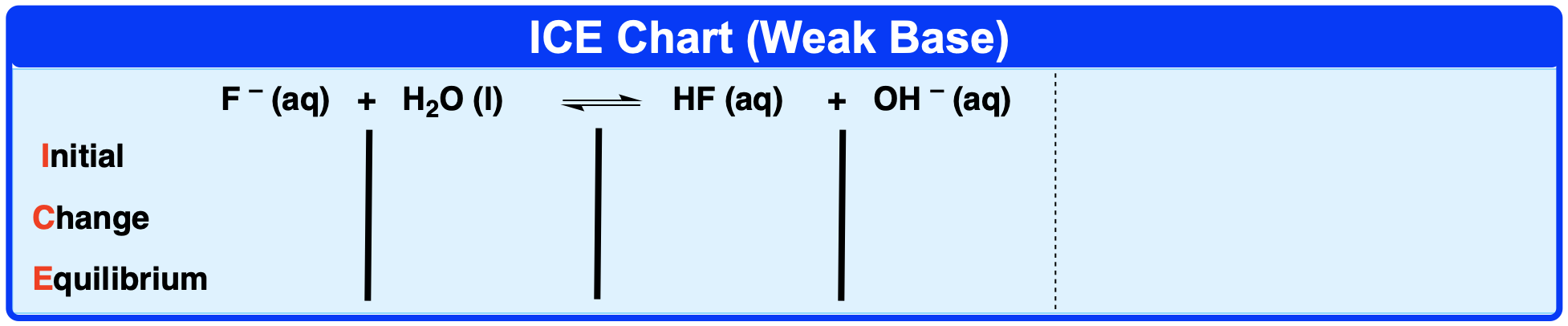Clutch Prep is now a part of Pearson
Ch.15 - Acid and Base EquilibriumWorksheetSee all chapters

# pH of Weak Bases

See all sections
Sections
Acids Introduction
Bases Introduction
Binary Acids
Oxyacids
Bases
Amphoteric Species
Arrhenius Acids and Bases
Bronsted-Lowry Acids and Bases
Lewis Acids and Bases
The pH Scale
Auto-Ionization
Ka and Kb
pH of Strong Acids and Bases
Ionic Salts
pH of Weak Acids
pH of Weak Bases
Diprotic Acids and Bases
Diprotic Acids and Bases Calculations
Triprotic Acids and Bases
Triprotic Acids and Bases Calculations
Conjugate Acids and Bases

Weak Bases partially ionize or dissociate when placed in a solution

###### Calculating pH

Concept #1: Weak Bases require an ICE Chart to determine their pH.

Example #1: Calculate the hydroxide ion concentration of a 0.55 M KF solution at 25ºC. The acid dissociation constant of HF is 3.5 x 10-4.

Example #2: What is the pH of a 0.12 M ethylamine, C2H5NH2, solution? The Kb value of ethylamine is 5.6 x 10-4.Concept #2: Weak Bases are weak electrolytes and so will ionize less than 100%.

Example #3: Calculate the percent ionization when 73.2 g sodium hypoiodite, NaIO, are dissolved with 500 mL of solution. The Ka value of hypoiodous acid, HIO, is 2.3 x 10-11.

Practice: Determine the pH of a solution made by dissolving 6.1 g of sodium cyanide, NaCN, in enough water to make a 500.0 mL of solution. (MW of NaCN = 49.01 g/mol). The Ka value of HCN is 4.9 × 1010.

Practice: An unknown weak base has an initial concentration of 0.750 M with a pH of 8.03. Calculate its equilibrium base constant.